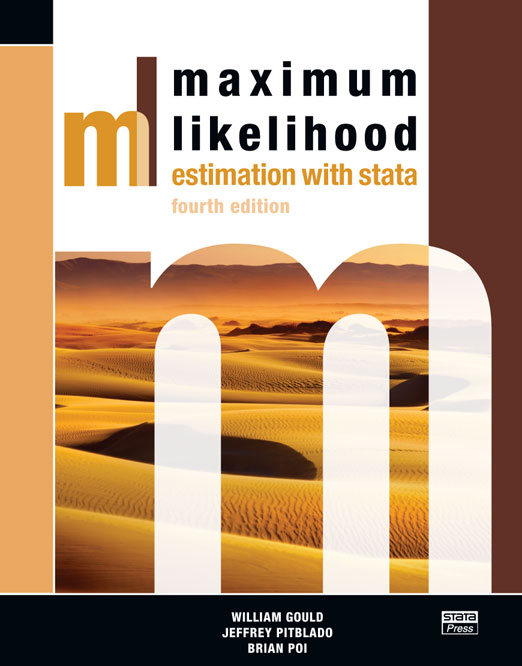# Maximum Likelihood Estimation with Stata

Maximum Likelihood Estimation with Stata, Fourth Edition is the essential reference and guide for researchers in all disciplines who wish to write maximum likelihood (ML) estimators in Stata. Beyond providing comprehensive coverage of Stata’s ml command for writing ML estimators, the book presents an overview of the underpinnings of maximum likelihood and how to think about ML estimation.

The book shows you how to take full advantage of the ml command’s noteworthy features:

linear constraints

four optimization algorithms (Newton–Raphson, DFP, BFGS, and BHHH)

observed information matrix (OIM) variance estimator

outer product of gradients (OPG) variance estimator

Huber/White/sandwich robust variance estimator

cluster–robust variance estimator

complete and automatic support for survey data analysis

direct support of evaluator functions written in Mata

When appropriate options are used, many of these features are provided automatically by ml and require no special programming or intervention by the researcher writing the estimator.

The fourth edition has been updated to include new features introduced in recent versions of Stata. Such features include new methods for handling scores, more consistent arguments for likelihood-evaluator programs, and support for likelihood evaluators written in Mata (Stata’s matrix programming language). The authors illustrate how to write your estimation command so that it fully supports factor-variable notation and the svy prefix for estimation with survey data. They have also restructured the chapters that introduce ml in a way that allows you to begin working with ml faster. This edition is essential for anyone using Stata 11.

In the final chapter, the authors illustrate the major steps required to get from log-likelihood function to fully operational estimation command. This is done using several different models: logit and probit, linear regression, Weibull regression, the Cox proportional hazards model, random-effects regression, and seemingly unrelated regression.

The authors provide extensive advice for developing your own estimation commands. With a little care and the help of this book, users will be able to write their own estimation commands—commands that look and behave just like the official estimation commands in Stata.

Whether you want to fit a special ML estimator for your own research or wish to write a general-purpose ML estimator for others to use, you need this book.

List of Tables
List of Figures
Preface to the fourth edition
Notation and typography
Versions of Stata

1. THEORY AND PRACTICE

The likelihood maximization problem
Likelihood theory

All results are asymptotic
Variance estimates and hypothesis tests
Likelihood-ratio tests and Wald tests
The outer product of gradients variance estimator
Robust variance estimates

The maximization problem

Numerical root finding

Newton’s method
The Newton-Raphson algorithm

Quasi-Newton methods

The BHHH algorithm
The DFP and BFGS algorithms

Numerical maximization
Numerical derivatives
Numerical second derivatives

Monitoring convergence

2. INTRODUCTION TO OF ml

The probit model
Normal linear regression
Robust standard errors
Weighted estimation
Other features of method-gf0 evaluators
Limitations

3. OVERVIEW OF ml

The terminology of ml
Equations in ml
Likelihood-evaluator methods
Tools for the ml programmer
Common ml options

Subsamples
Weights
OPG estimates of variance
Robust estimates of variance
Survey data
Constraints
Choosing among the optimization algorithms

4. METHODS lf

The linear-form restrictions
Examples

The probit model
Normal linear regression
The Weibull model

The importance of generating temporary variables as doubles
Problems you can safely ignore
Nonlinear specifications
The advantages of lf in terms of execution speed

5. METHODS lf0, lf1, AND lf2

Comparing these methods
Outline of evaluators of methods lf0, lf1, and lf2

The todo argument
The b argument

Using mleval to obtain values from each equation

The lnfj argument
Arguments for scores
The H argument

Using mlmatsum to define H

Aside: Stata’s scalars

Summary of methods lf0, lf1, and lf2

Method lf0
Method lf1
Method lf2

Examples

The probit model
Normal linear regression
The Weibull model

6. METHODS d0, d1, AND d2

Comparing these methods
Outline of method d0, d1, and d2 evaluators

The todo argument
The b argument
The lnf argument

Using lnf to indicate that the likelihood cannot be calculated
Using mlsum to define lnf

The g argument

Using mlvecsum to define g

The H argument

Summary of methods d0, d1, and d2

Method d0
Method d1
Method d2

Panel-data likelihoods

Calculating lnf
Calculating g
Calculating H

Using mlmatbysum to help define H

Other models that do not meet the linear-form restrictions

7. DEBUGGING LIKELIHOOD EVALUATORS

ml check
Using the debug methods

First derivatives
Second derivatives

ml trace

8. SETTING INITIAL VALUES

ml search
ml plot
ml init

9. INTERACTIVE MAXIMIZATION

The iteration log
Pressing the Break key
Maximizing difficult likelihood functions

10. FINAL RESULTS

Graphing convergence
Redisplaying output

11. MATA-BASED LIKELIHOOD EVALUATORS

Introductory examples

The probit model
The Weibull model

Evaluator function prototypes

Method-lf evaluators
lf-family evaluators
d-family evaluators

Utilities

Dependent variables
Obtaining model parameters
Summing individual or group-level log likelihoods
Calculating the Hessian

Random-effects linear regression

Calculating lnf
Calculating g
Calculating H
Results at last

12. WRITING DO-FILES TO MAXIMIZE LIKELIHOODS

The structure of a do-file
Putting the do-file into production

13. WRITING ADO-FILES TO MAXIMIZE LIKELIHOODS

Writing estimation commands
The standard estimation-command outline
Outline for estimation commands using ml
Using ml in noninteractive mode

Syntax
Estimation subsample
Parsing with help from mlopts
Weights
Constant-only model
Initial values
Saving results in e()
Displaying ancillary parameters
Exponentiated coefficients
Offsetting linear equations
Program properties

14. WRITING ADO-FILES FOR SURVEY DATA ANALYSIS

Program properties

15. OTHER EXAMPLES

The logit model
The probit model
Normal linear regression
The Weibull model
The Cox proportional hazards model
The random-effects regression model
The seemingly unrelated regression model

A. SYNTAX OF ML

B. LIKELIHOOD-EVALUATOR CHECKLISTS

Method lf
Method d0
Method d1
Method d2
Method lf0
Method lf1
Method lf2

C. LISTING OF ESTIMATION COMMANDS

The logit model
The probit model
The normal model
The Weibull model
The Cox proportional hazards model
The random-effects regression model
The seemingly unrelated regression modelAuthor: William Gould, Jeffrey Pitblado and Brian Poi
Edition: Fourth Edition
ISBN-13: 978-1-59718-078-8